# RS Aggarwal Solutions for Class 7 Maths Chapter 18 Reflection and Rotational Symmetry

RS Aggarwal Solutions for Class 7 Maths Chapter 18 – Reflection and Rotational Symmetry is the best study material, which provides solutions to all the questions present in the RS Aggarwal class 7 textbook. Students can refer to these solutions and practise various questions daily to perform well in the final exams. Our experts prepare these study materials as per the CBSE syllabus. Students who aspire to score good marks in Maths, then refer RS Aggarwal Class 7 Solutions.

Expert tutors at BYJU’S have prepared the RS Aggarwal Solutions for Class 7 Maths Chapter 18, and it contains all exercises. Students gain more knowledge by referring to RS Aggarwal Solutions. Download pdf of Class 7 Chapter 18 in their respective links.

## Download PDF of RS Aggarwal Solutions for Class 7 Maths Chapter 18 – Reflection and Rotational Symmetry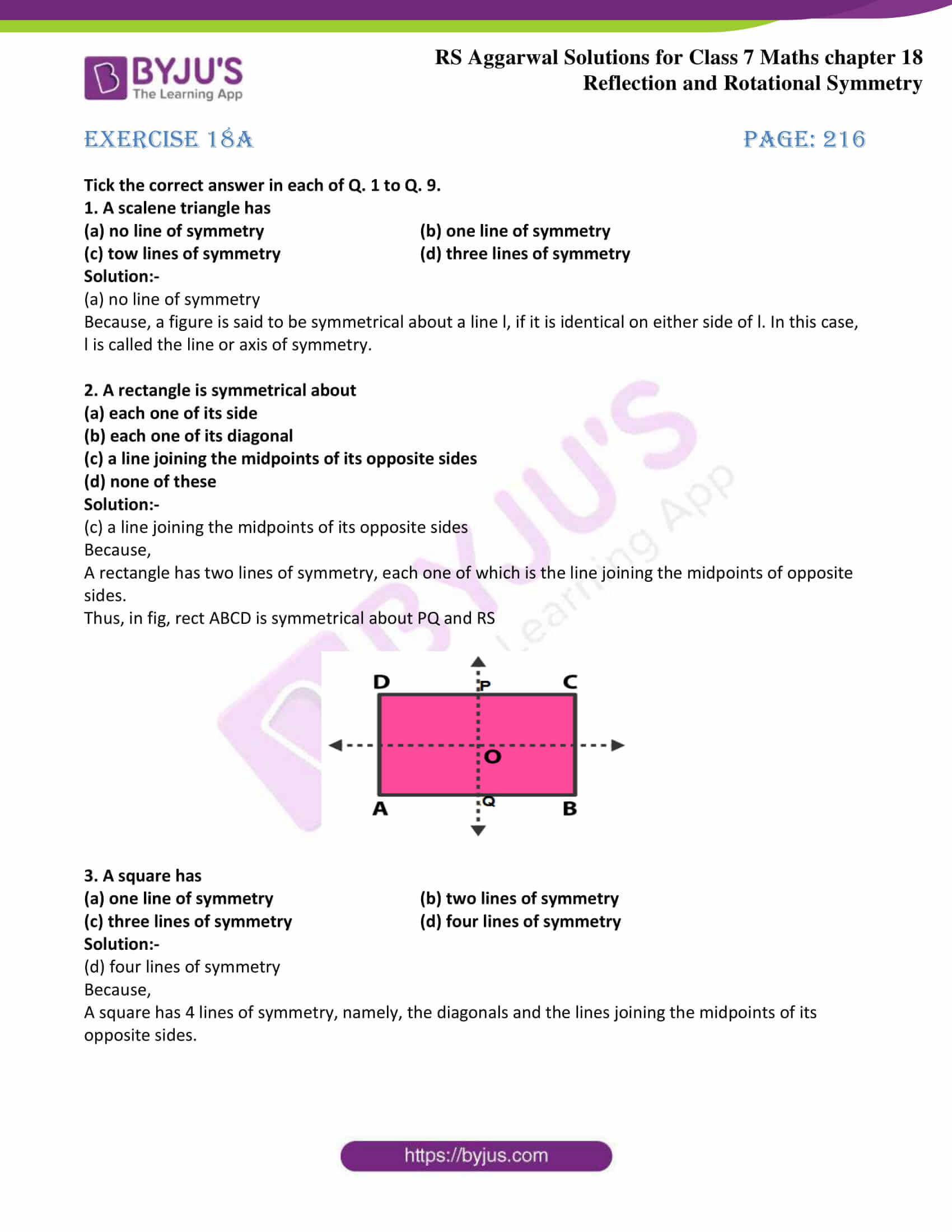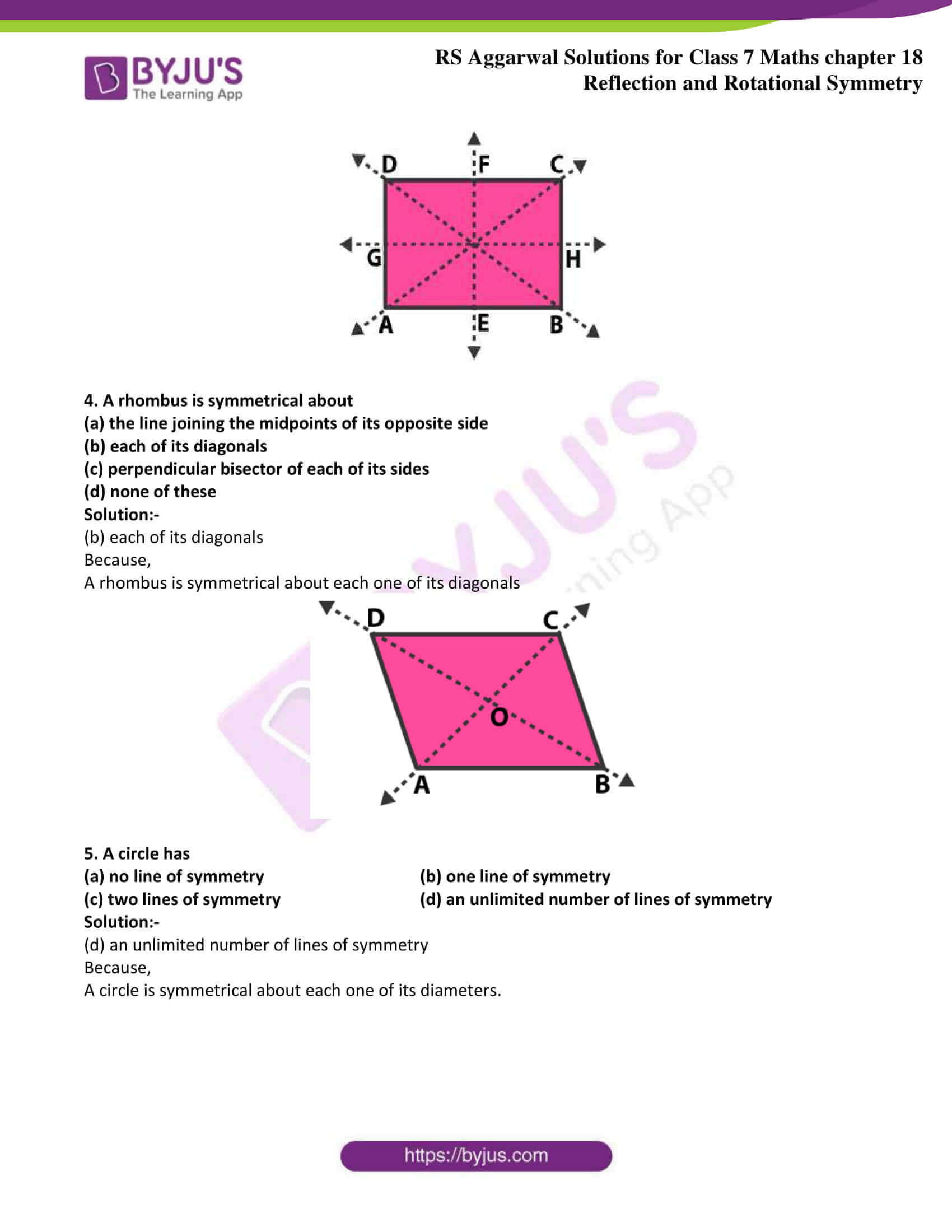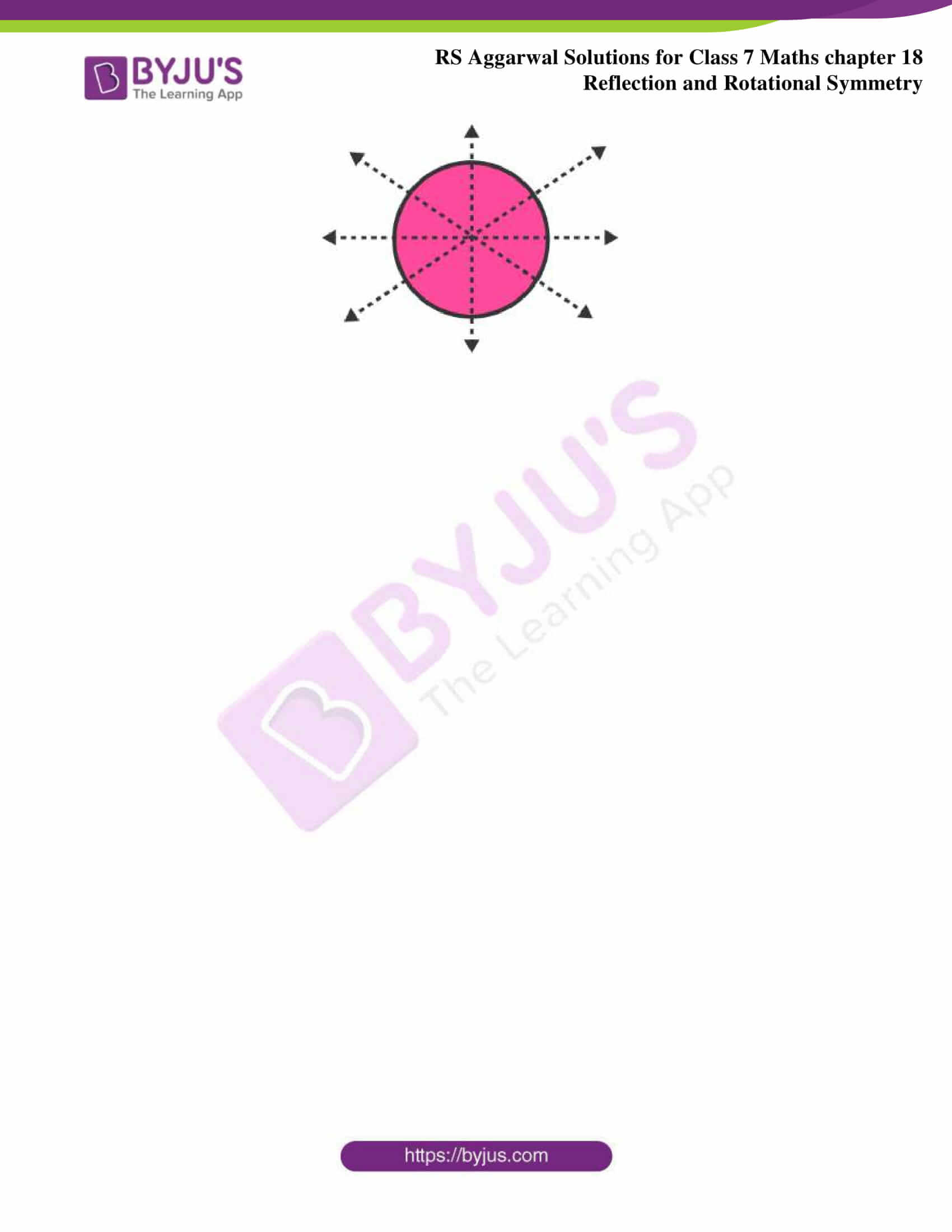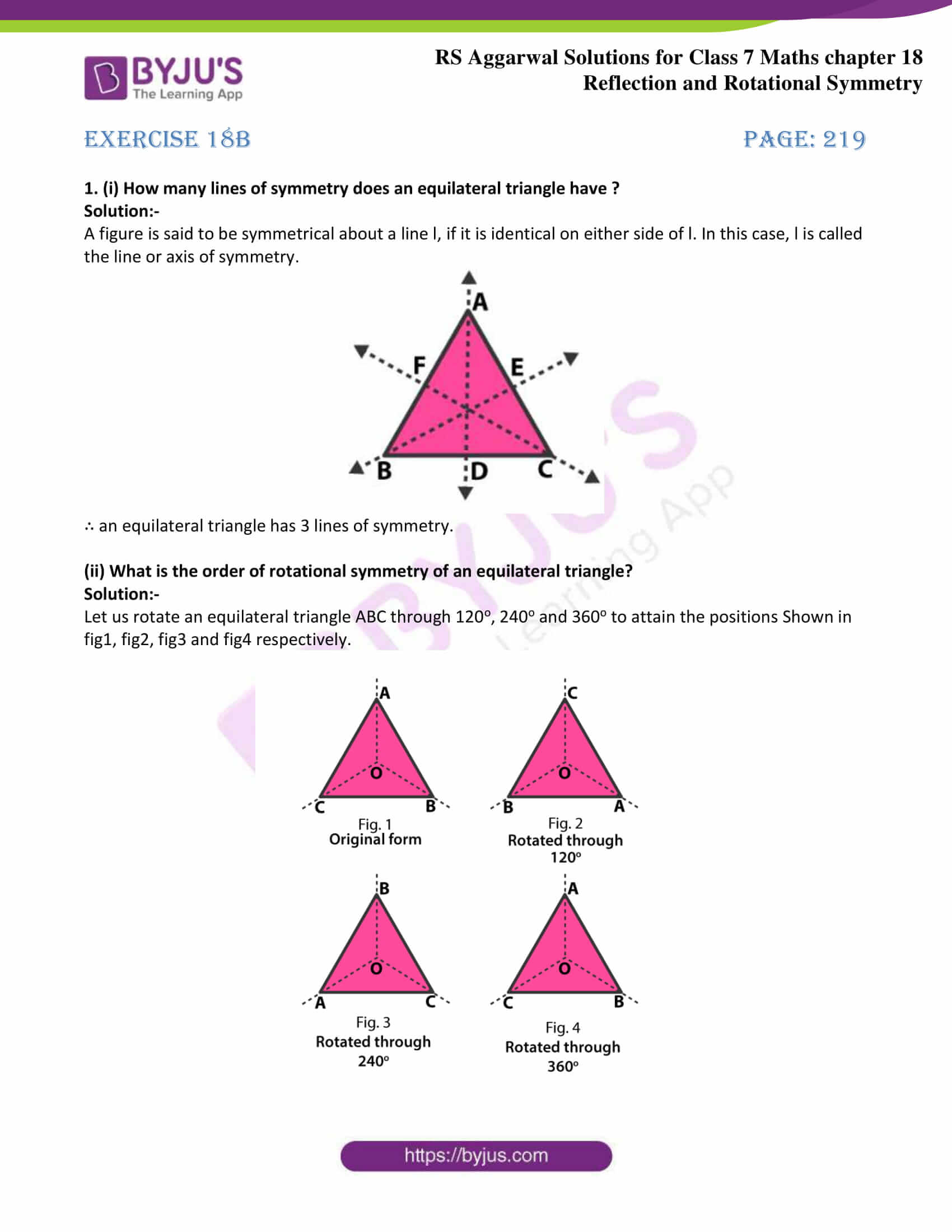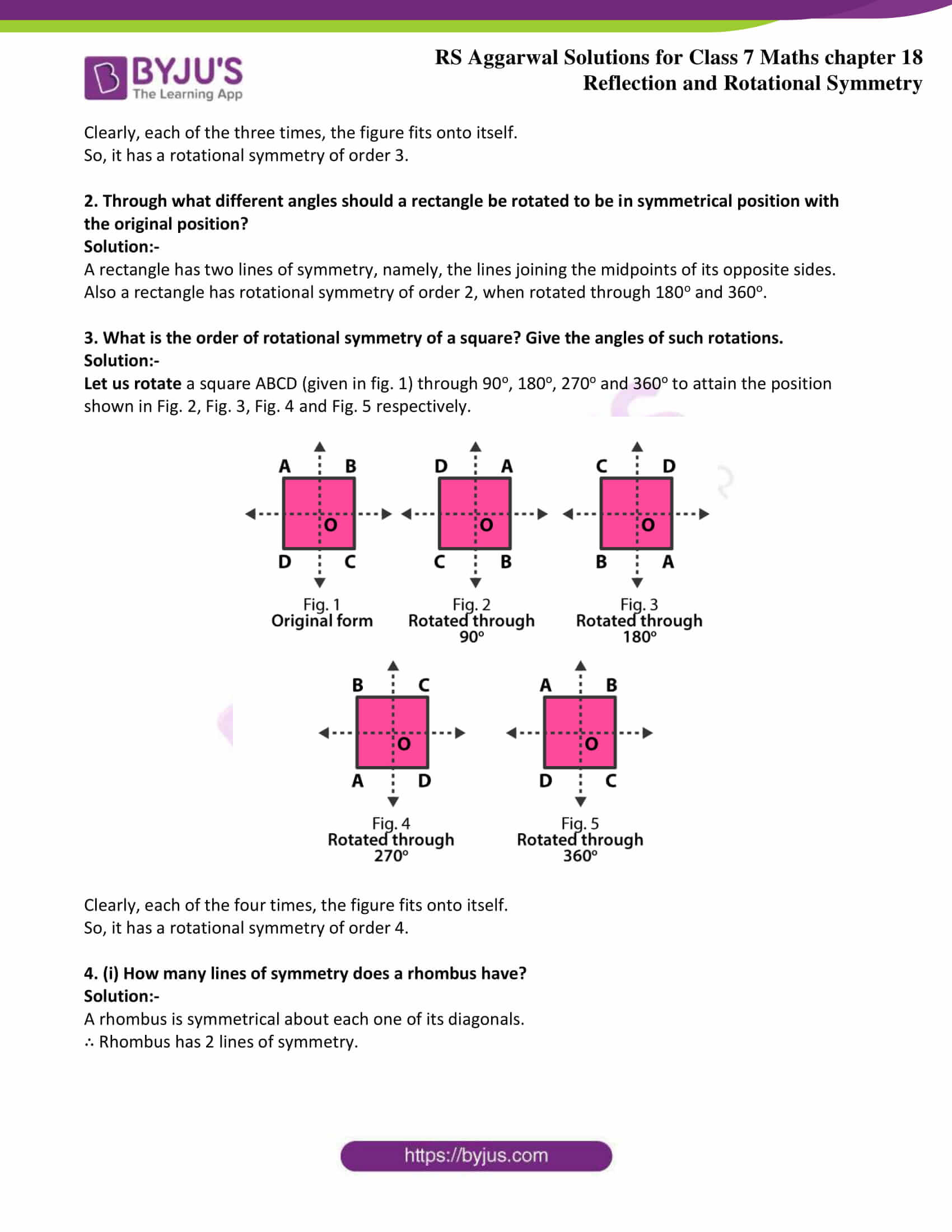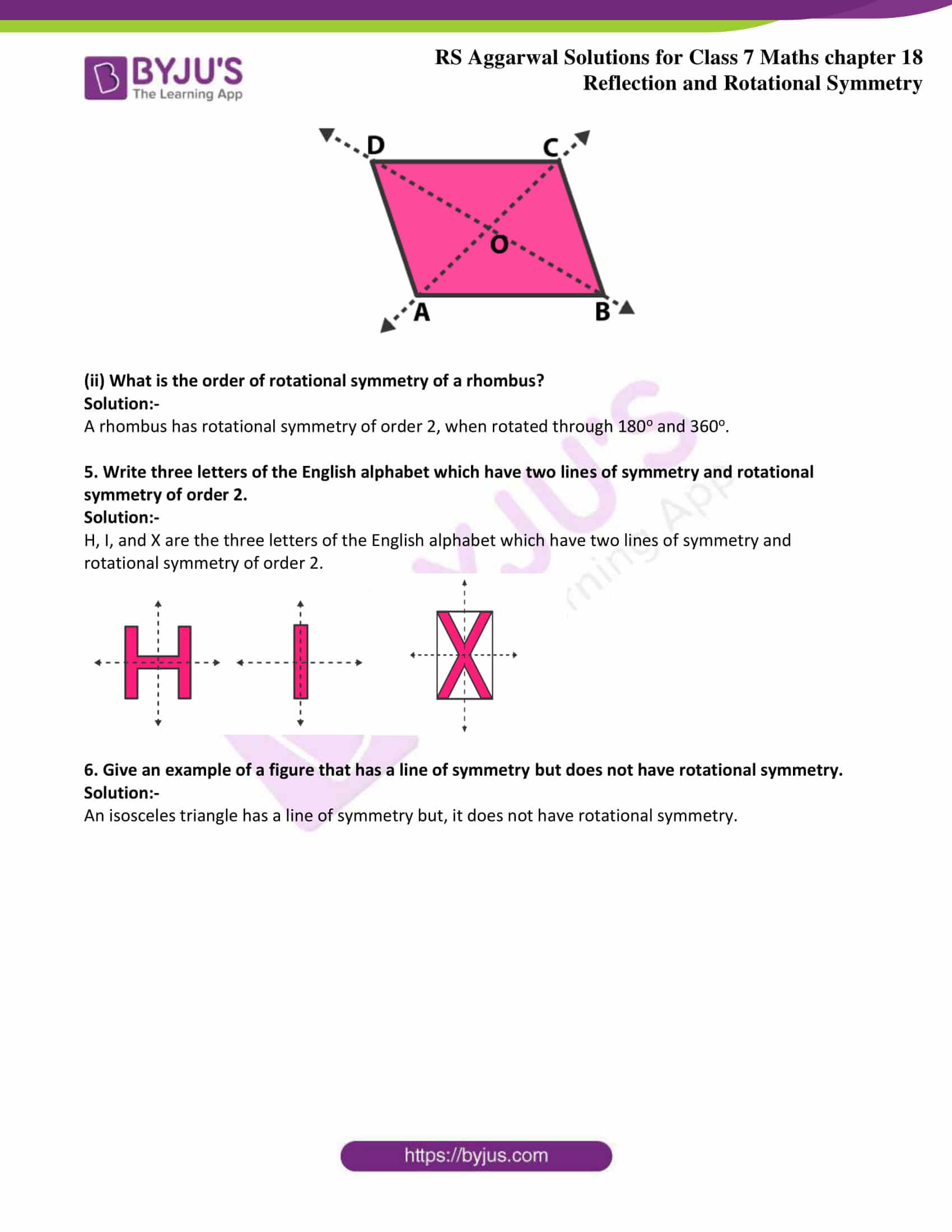Exercise 18A

Exercise 18B

## Exercise 18A Page: 216

Tick the correct answer in each of Q. 1 to Q. 9.

1. A scalene triangle has

(a) no line of symmetry (b) one line of symmetry

(c) tow lines of symmetry (d) three lines of symmetry

Solution:-

(a) no line of symmetry

Because, a figure is said to be symmetrical about a line l, if it is identical on either side of l. In this case, l is called the line or axis of symmetry.

2. A rectangle is symmetrical about

(a) each one of its side

(b) each one of its diagonal

(c) a line joining the midpoints of its opposite sides

(d) none of these

Solution:-

(c) a line joining the midpoints of its opposite sides

Because,

A rectangle has two lines of symmetry, each one of which is the line joining the midpoints of opposite sides.

Thus, in fig, rect ABCD is symmetrical about PQ and RS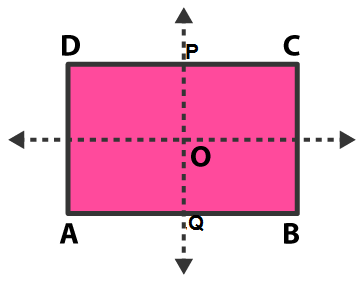3. A square has

(a) one line of symmetry (b) two lines of symmetry

(c) three lines of symmetry (d) four lines of symmetry

Solution:-

(d) four lines of symmetry

Because,

A square has 4 lines of symmetry, namely, the diagonals and the lines joining the midpoints of its opposite sides.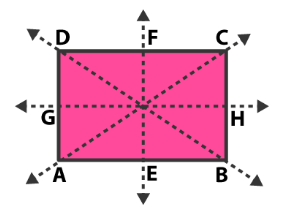4. A rhombus is symmetrical about

(a) the line joining the midpoints of its opposite side

(b) each of its diagonals

(c) perpendicular bisector of each of its sides

(d) none of these

Solution:-

(b) each of its diagonals

Because,

A rhombus is symmetrical about each one of its diagonals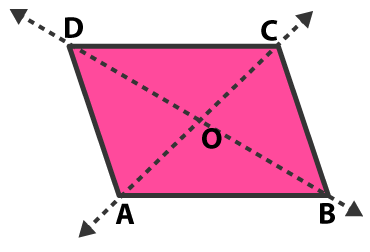5. A circle has

(a) no line of symmetry (b) one line of symmetry

(c) two lines of symmetry (d) an unlimited number of lines of symmetry

Solution:-

(d) an unlimited number of lines of symmetry

Because,

A circle is symmetrical about each one of its diameters.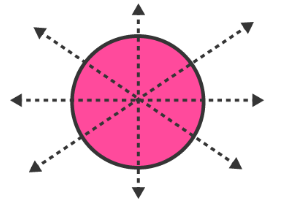## Exercise 18B Page: 219

1. (i) How many lines of symmetry does an equilateral triangle have ?

Solution:-

A figure is said to be symmetrical about a line l, if it is identical on either side of l. In this case, l is called the line or axis of symmetry.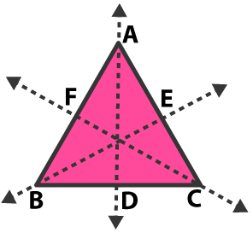∴ an equilateral triangle has 3 lines of symmetry.

(ii) What is the order of rotational symmetry of an equilateral triangle?

Solution:-

Let us rotate an equilateral triangle ABC through 120o, 240o and 360o to attain the positions Shown in fig1, fig2, fig3 and fig4 respectively.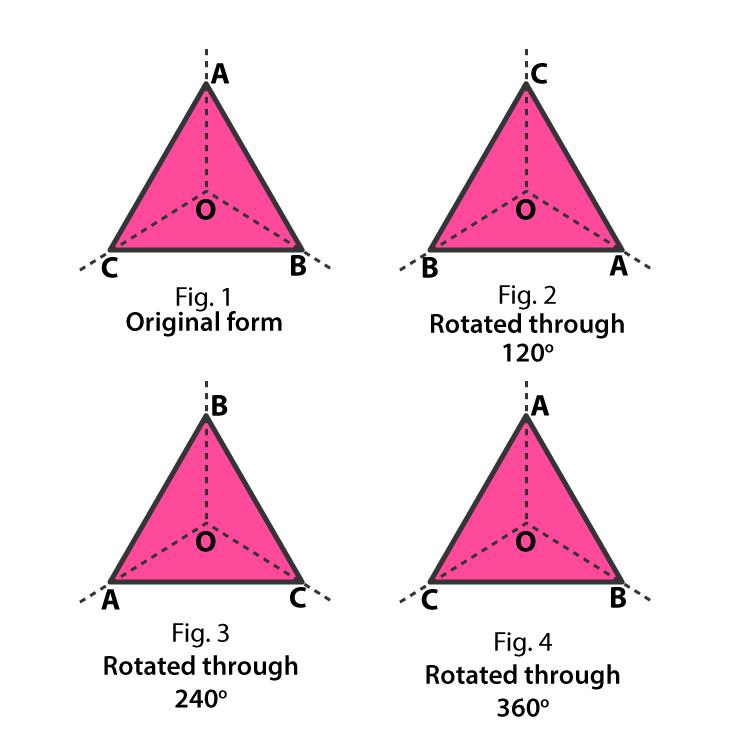Clearly, each of the three times, the figure fits onto itself.

So, it has a rotational symmetry of order 3.

2. Through what different angles should a rectangle be rotated to be in symmetrical position with the original position?

Solution:-

A rectangle has two lines of symmetry, namely, the lines joining the midpoints of its opposite sides.

Also a rectangle has rotational symmetry of order 2, when rotated through 180o and 360o.

3. What is the order of rotational symmetry of a square? Give the angles of such rotations.

Solution:-

Let us rotate a square ABCD (given in fig. 1) through 90o, 180o, 270o and 360o to attain the position shown in Fig. 2, Fig. 3, Fig. 4 and Fig. 5 respectively.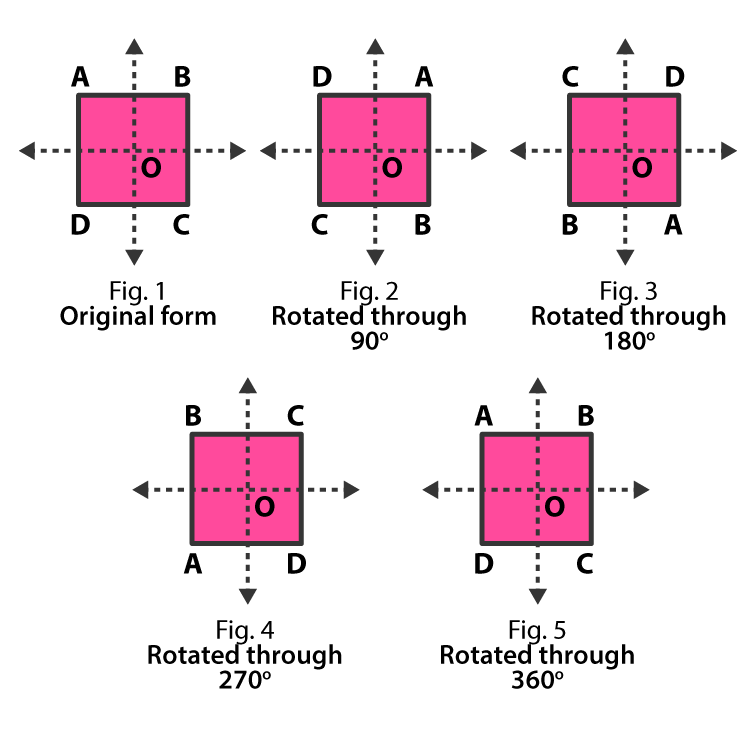Clearly, each of the four times, the figure fits onto itself.

So, it has a rotational symmetry of order 4.

4. (i) How many lines of symmetry does a rhombus have?

Solution:-

A rhombus is symmetrical about each one of its diagonals.

∴ Rhombus has 2 lines of symmetry.(ii) What is the order of rotational symmetry of a rhombus?

Solution:-

A rhombus has rotational symmetry of order 2, when rotated through 180o and 360o.

5. Write three letters of the English alphabet which have two lines of symmetry and rotational symmetry of order 2.

Solution:-

H, I, and X are the three letters of the English alphabet which have two lines of symmetry and rotational symmetry of order 2.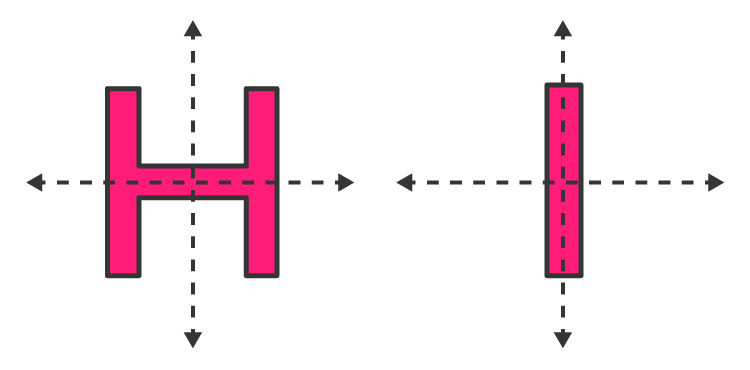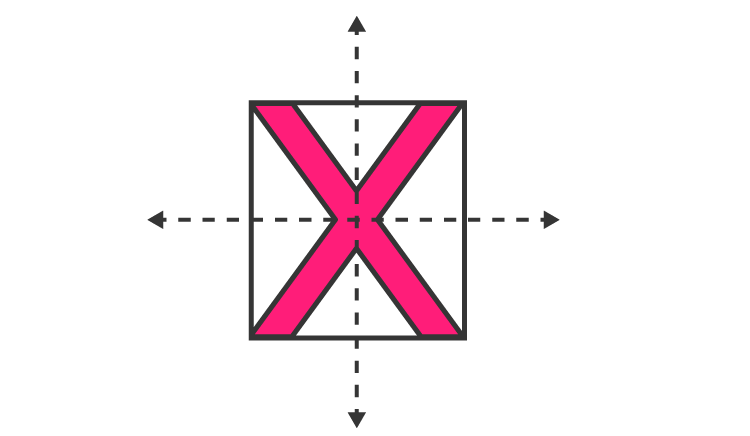6. Give an example of a figure that has a line of symmetry but does not have rotational symmetry.

Solution:-

An isosceles triangle has a line of symmetry but, it does not have rotational symmetry.

## RS Aggarwal Solutions for Class 7 Maths Chapter 18 – Reflection and Rotational Symmetry

Chapter 18 – Reflection and Rotational Symmetry contains 2 exercises and the RS Aggarwal Solutions available on this page provide solutions to the questions present in the exercises. Now, let us have a look at some of the concepts discussed in this chapter.

• Reflection Symmetry
• Rotational Symmetry
• Figures Having Line Symmetry and Rotational Symmetry

### Chapter Brief of RS Aggarwal Solutions for Class 7 Maths Chapter 18 – Reflection and Rotational Symmetry

RS Aggarwal Solutions for Class 7 Maths Chapter 18 – Reflection and Rotational Symmetry. A figure is said to be symmetrical about a line l, if it is identical on either side of l. In this case, l is called the line or axis of symmetry. A figure is said to have rotational symmetry if it fits onto itself more than once during a complete rotation.